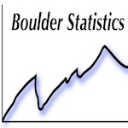Choose Language Hide Translation Bar

## Number line

How do I create a simple elementary school type number line in JMP?  I'd like to plot numbers such as 0.8, 0.9, 0.92, 0.94 on a number line.  I've created a chart, using data statistics with the horizontal & point chart options--how do I get rid of the Y axis so the values will be plotted on the X axis only?

1 ACCEPTED SOLUTION

Accepted Solutions
HighlightedStaffJoined:

Mar 21, 2013

Solution

## Re: Number line

same idea, using JSL.  Looks sharp if the graph background matches the window background.

Making Anonymous Graphs in JMP 8 - JMP Blog has some secrets for removing extra lines and text from a graph, reused here.

JMP 11 lets the vertical axis be very short, which helps the appearance.  Two reference lines, both at zero, look like axes.`dt = New Table( "Untitled", New Column( "x", Set Values( [0.1, 0.8, 0.7, 0.3, 0.4] ) ) );xvals = dt:x << get values;yvals = J( N Rows( xvals ), 1, 0 );New Window( "Number Line",    BorderBox(left(10),top(10),right(10),bottom(10), vlistbox(    Text Box( "Points on a number line" ),    Spacer Box( size( 10, 50 ) ),    g = Graph Box( Frame Size( 500, 7 ),        X Scale( -0.1, 1.1 ), Y Scale( -1, 1 ),        yname( " " ), Marker Size( 2 ),         yaxis( show major ticks( false ), show minor ticks( false ),            show labels( false ), Inside Ticks( 1 ),            Add Ref Line( 0.0, Solid, "Black", "", 1 ) ),        xaxis( show major ticks( false ), show minor ticks( false ),            show labels( true ), Add Ref Line( 0.0, Solid, "Black", "", 1 ),            Inside Ticks( 1 ) ),        Marker( Combine States( Marker State( 12 ),color state("red") ), xvals, yvals )    ),    Spacer Box( size( 10, 50 ) ),    Text Box( "drag with the axis" )    )));// http://blogs.sas.com/content/jmp/2008/11/10/making-anonymous-graphs-in-jmp-8/g[axis box( 1 )] << remove axis label;g[axis box( 2 )] << remove axis label;// hide the inner borderg[frame box( 1 )] << Left( 0 ); // no line on left, etcg[frame box( 1 )] << Right( 0 );g[frame box( 1 )] << top( 0 );g[frame box( 1 )] << bottom( 0 );// shrink and hide the outer border toog[Border Box( 1 )] << Left( 0 ); // no space on left, etcg[Border Box( 1 )] << Right( 0 );g[Border Box( 1 )] << Top( 0 );g[Border Box( 1 )] << bottom( 0 );g[Border Box( 1 )] << sides( 0 ); // draw no sides (1+2+4+8 would be all four sides)`
Craige
2 REPLIES 2Super UserJoined:

Feb 10, 2013

## Re: Number line

Try graph builder, just drag your column to the x-axis.HighlightedStaffJoined:

Mar 21, 2013

Solution

## Re: Number line

same idea, using JSL.  Looks sharp if the graph background matches the window background.

Making Anonymous Graphs in JMP 8 - JMP Blog has some secrets for removing extra lines and text from a graph, reused here.

JMP 11 lets the vertical axis be very short, which helps the appearance.  Two reference lines, both at zero, look like axes.`dt = New Table( "Untitled", New Column( "x", Set Values( [0.1, 0.8, 0.7, 0.3, 0.4] ) ) );xvals = dt:x << get values;yvals = J( N Rows( xvals ), 1, 0 );New Window( "Number Line",    BorderBox(left(10),top(10),right(10),bottom(10), vlistbox(    Text Box( "Points on a number line" ),    Spacer Box( size( 10, 50 ) ),    g = Graph Box( Frame Size( 500, 7 ),        X Scale( -0.1, 1.1 ), Y Scale( -1, 1 ),        yname( " " ), Marker Size( 2 ),         yaxis( show major ticks( false ), show minor ticks( false ),            show labels( false ), Inside Ticks( 1 ),            Add Ref Line( 0.0, Solid, "Black", "", 1 ) ),        xaxis( show major ticks( false ), show minor ticks( false ),            show labels( true ), Add Ref Line( 0.0, Solid, "Black", "", 1 ),            Inside Ticks( 1 ) ),        Marker( Combine States( Marker State( 12 ),color state("red") ), xvals, yvals )    ),    Spacer Box( size( 10, 50 ) ),    Text Box( "drag with the axis" )    )));// http://blogs.sas.com/content/jmp/2008/11/10/making-anonymous-graphs-in-jmp-8/g[axis box( 1 )] << remove axis label;g[axis box( 2 )] << remove axis label;// hide the inner borderg[frame box( 1 )] << Left( 0 ); // no line on left, etcg[frame box( 1 )] << Right( 0 );g[frame box( 1 )] << top( 0 );g[frame box( 1 )] << bottom( 0 );// shrink and hide the outer border toog[Border Box( 1 )] << Left( 0 ); // no space on left, etcg[Border Box( 1 )] << Right( 0 );g[Border Box( 1 )] << Top( 0 );g[Border Box( 1 )] << bottom( 0 );g[Border Box( 1 )] << sides( 0 ); // draw no sides (1+2+4+8 would be all four sides)`
Craige